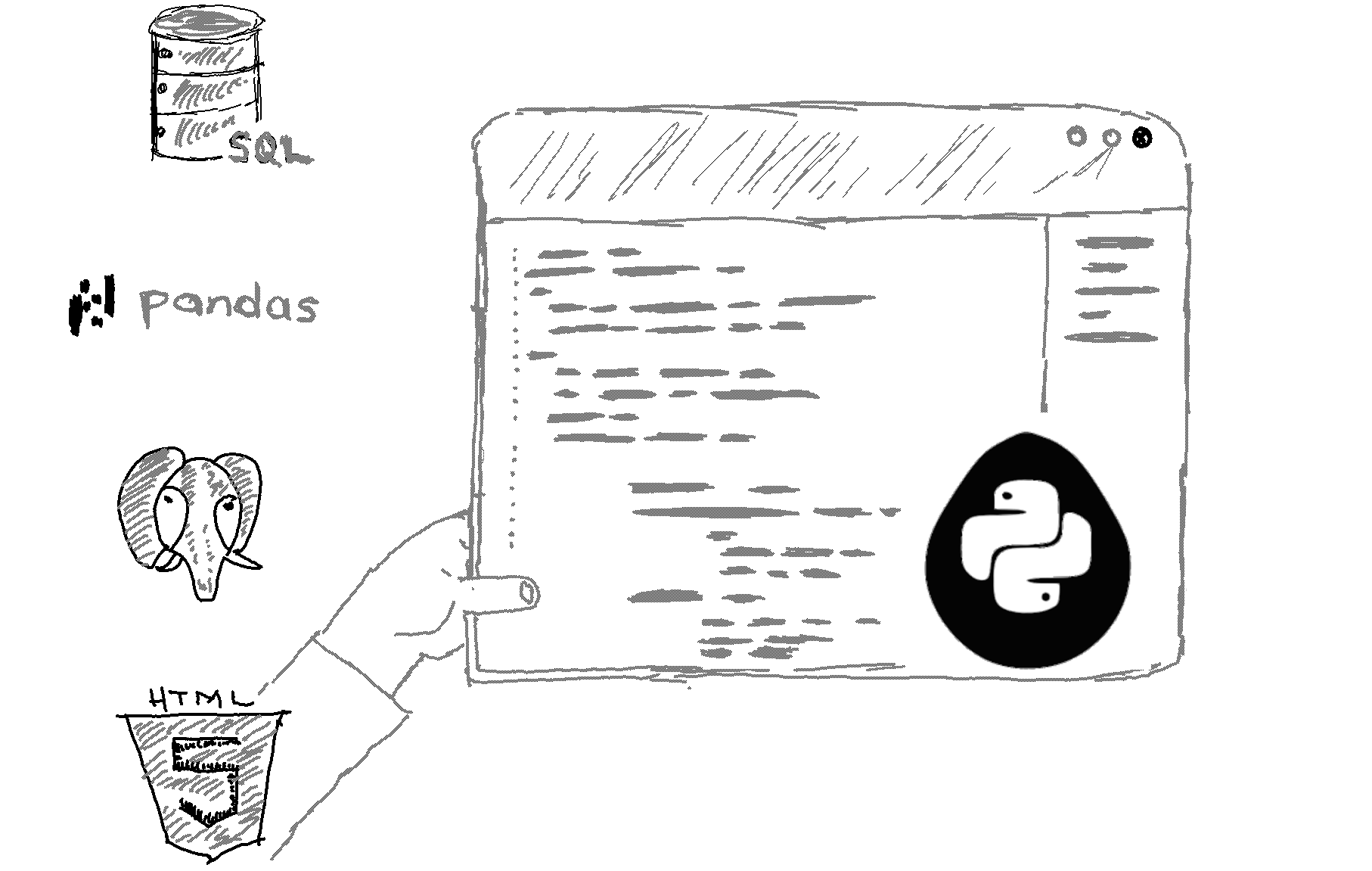# Here is how to sort a dictionary by value in Python.

```# Define a dictionary
my_dict = {
"key1": "value1",
"key2": "value2",
"key3": "value3"
}

# Sort the dictionary by value
sorted_dict = sorted(my_dict.items(), key=lambda x: x)

# Print the sorted dictionary
print(sorted_dict) ```

Output
`[("key1", "value1"), ("key2", "value2"), ("key3", "value3")]`

Explanation

To sort a dictionary by value in Python, you can use the sorted() function and pass it a lambda function that returns the value from each key-value pair.

As you can see, the dictionary has been sorted in ascending order based on the values.

Note that the sorted() function returns a list of tuples, where each tuple contains a key-value pair from the dictionary. If you want to get a dictionary back instead of a list of tuples, you can use the dict() function to convert the list of tuples to a dictionary, like this:

```# Define a dictionary
my_dict = {
"key1": "value1",
"key2": "value2",
"key3": "value3"
}

# Sort the dictionary by value
sorted_dict = dict(sorted(my_dict.items(), key=lambda x: x))

# Print the sorted dictionary
print(sorted_dict)```
This will print the following:

`{"key1": "value1", "key2": "value2", "key3": "value3"}`
As you can see, the dictionary has been sorted in ascending order based on the values and then converted back to a dictionary.

Related HowTos
##### Recommended Course

Learn Flask development and learn to build cool apps with our premium Python course on Udemy.